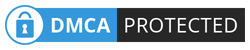The accommodation of various odds and bets in Roulette has seen various gameplay strategies adopted over the years. Among the most popular ones that have stood out to be used around the world in different variations is the Fibonacci System. This mode of gameplay is as popular as others like the Martingale System, but it comes with a smaller risk.

The Fibonacci sequence is the brainchild of Leonardo Fibonacci who lived between 1170 and 1250. Leonardo was an acclaimed mathematician that is credited with introducing the Arabic numeral system to Europe, which replaced the Roman one. The Fibonacci number sequence lines up as one of the man’s achievements, which explains a lot of natural phenomena like the arrangement of flowers and pine cones. The system has also proved useful in the game of roulette, and its use, upsides, and cons are assessed in this review.

## Mathematics of Fibonacci Numbers

The Fibonacci sequence is a number string that is created by adding the previous two numbers in the system to create the next one. The numerals in this system have no limit and can be used to count as far up as one wishes. Below is a look of how the sequence works:

• One – the first number of the series
• One – a sum of the first number and zero
• Two – a total of one and one
• Three – a sum of two and one
• Five – two and three put together
• Eight – five and three added together
• Thirteen – a sum of eight and five, and so on.

This sequence is not only witnessed in gaming tables but also natural occurrences such as a bee family. The series can also be represented in the form of a rectangle where the length is the bigger number and width is the preceding smaller number. In the structure, tiny squares can be fitted that add up to the Fibonacci numbers used to get to its dimensions. The squares end up forming a spiral when a line is drawn to connect them, which resembles some natural patterns such as some snail shells or the order of flower petals in some plants.

## Fibonacci System Works

The application of the Fibonacci system in roulette cannot be traced back to a specific time, but gamblers have been using it in the game for a significant period. Like most other roulette betting systems, the Fibonacci strategy is built to work when used on even-money bets. This restriction is necessary due to the fact that these types of stakes usually have about a fifty-fifty per cent chance of being landed, which is the highest. Inside bets, however, have low odds of winning, which means there is a huge chance of incurring losses in the end.

The Fibonacci betting system just requires gamers to follow the number series to determine the stakes that they need to place until winnings are landed. Once this happens, it is advisable only to move back two steps and proceed from there. For example, when you begin with a stake of one dollar and win on the five-dollar stake, you will end up with three extra dollars. If you proceed with a two-dollar wager and win with the three-dollar bet, you will pocket a profit of eight dollars.

Therefore, even after six losses and two wins, a large profit margin is collected despite playing on safe terms for the most part. In the same number of rounds, 245 dollars would have to be spent when using the Martingale System, and the profit would only be a dollar. With Fibonacci, on the other hand, only 22 dollars are used.

## Pitfalls of Fibonacci System

Even though the Fibonacci system appears a lot safer than most others, it does have its downsides that still maintain the dependence of chance in the game of roulette. The mathematics sequence can end up being a flop when looking at various elements such as:

• Long-term use: Fibonacci strategy is similar to most others in the sense that it does not work over long periods. Considering it is a progressive betting system, one can reach the maximum staking limit before a win is landed. When this happens, punters can only count their loses and walk away or start from scratch. The limit can be that of the player or the casino.
• Unchanging odds: Even with the suggestions of the stakes to place, Fibonacci strategy does not change the ratio of landing wins. Roulette games depend on luck, which means losses are not eliminated from the table.

## Relatively Safe but Will Not Win You a Lot

The Fibonacci strategy is undoubtedly safer to apply than most others used in both old and new versions of roulette. Even so, it should only be used in moderation. Players should keep in mind that the house always has the upper hand, and chance is still used to determine winnings.